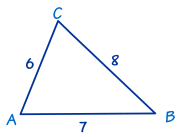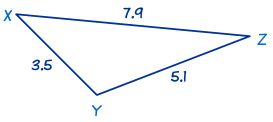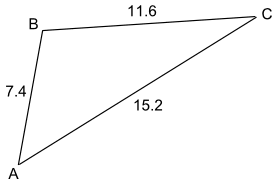# Solving SSS Triangles

"SSS" means "Side, Side, Side""SSS" is when we know three sides of the triangle, and want to find the missing angles.

 To solve an SSS triangle: use The Law of Cosines first to calculate one of the angles then use The Law of Cosines again to find another angle and finally use angles of a triangle add to 180° to find the last angle.

We use the "angle" version of the Law of Cosines:

cos(C) = a2 + b2 − c2 2ab

cos(A) = b2 + c2 − a2 2bc

cos(B) = c2 + a2 − b2 2ca

(they are all the same formula, just different labels)

### Example 1In this triangle we know the three sides:

• a = 8,
• b = 6 and
• c = 7.

Use The Law of Cosines first to find one of the angles. It doesn't matter which one. Let's find angle A first:

cos A = (b2 + c2 − a2) / 2bc
cos A = (62 + 72 − 82) / (2×6×7)
cos A = (36 + 49 − 64) / 84
cos A = 0.25
A = cos−1(0.25)
A = 75.5224...°
A = 75.5° to one decimal place.

Next we will find another side. We use The Law of Cosines again, this time for angle B:

cos B = (c2 + a2 − b2)/2ca
cos B = (72 + 82 − 62)/(2×7×8)
cos B = (49 + 64 − 36) / 112
cos B = 0.6875
B = cos−1(0.6875)
B = 46.5674...°
B = 46.6° to one decimal place

Finally, we can find angle C by using 'angles of a triangle add to 180°':

C = 180° − 75.5224...° − 46.5674...°
C = 57.9° to one decimal place

Now we have completely solved the triangle i.e. we have found all its angles.

The triangle can have letters other than ABC:

### Example 2This is also an SSS triangle.

In this triangle we know the three sides x = 5.1, y = 7.9 and z = 3.5. Use The Law of Cosines to find angle X first:

cos X = (y2 + z2 − x2)/2yz
cos X = ((7.9)2 + (3.5)2 − (5.1)2)/(2×7.9×3.5)
cos X = (62.41 + 12.25 − 26.01)/55.3
cos X = 48.65/55.3 = 0.8797...
X = cos−1(0.8797...)
X = 28.3881...°
X = 28.4° to one decimal place

Next we will use The Law of Cosines again to find angle Y:

cos Y = (z2 + x2 − y2)/2zx
cos Y = −24.15/35.7 = −0.6764...
cos Y = (12.25 + 26.01 − 62.41)/35.7
cos Y = −24.15/35.7 = −0.6764...
Y = cos−1(−0.6764...)
Y = 132.5684...°
Y = 132.6° to one decimal place.

Finally, we can find angle Z by using 'angles of a triangle add to 180°':

Z = 180° − 28.3881...° − 132.5684...°
Z = 19.0° to one decimal place

## Another Method

 Here is another (slightly faster) way to solve an SSS triangle: use The Law of Cosines first to calculate the largest angle then use The Law of Sines to find another angle and finally use angles of a triangle add to 180° to find the last angle.

### Largest Angle?

Why do we try to find the largest angle first? That way the other two angles must be acute (less than 90°) and the Law of Sines will give correct answers.

The Law of Sines is difficult to use with angles above 90°. There can be two answers either side of 90° (example: 95° and 85°), but a calculator will only give you the smaller one.

So by calculating the largest angle first using the Law of Cosines, the other angles are less than 90° and the Law of Sines can be used on either of them without difficulty.

### Example 3B is the largest angle, so find B first using the Law of Cosines:

cos B = (a2 + c2 − b2) / 2ac
cos B = (11.62 + 7.42 − 15.22) / (2×11.6×7.4)
cos B = (134.56 + 54.76 − 231.04) / 171.68
cos B = −41.72 / 171.68
cos B = −0.2430...
B = 104.1° to one decimal place

Use the Law of Sines, sinC/c = sinB/b, to find angle A:

sin C / 7.4 = sin 104.1° / 15.2
sin C = 7.4 × sin 104.1° / 15.2
sin C = 0.4722...
C = 28.2° to one decimal place

Find angle A using "angles of a triangle add to 180":

A = 180° − (104.1° + 28.2°)
A = 180° − 132.3°
A = 47.7° to one decimal place

So A = 47.7°, B = 104.1°, and C = 28.2°